# Lake Balaton Laser experiment to determine the curvature of the Earth, if any.

Status
Not open for further replies.

#### Mick West

Staff member
Thanks for the calculation. We will provide the raw data to YOU in first hand to match our findings.
We are making it in AutoCad and showing you the results as well.

"1 foot/mile laser slope"
By this you mean that the laser should RISE about 1 foot / mile on the distance? roughly?
Can you make an expected laser beam height above water surface calculation at 5, 10 and 15 miles distance?

I am happy that we agree on the subject of leveling the laser! VERY important.

No, the point is you CAN'T level the laser to the degree of accuracy needed. Hence you need to factor that into the calculations (as I did above).

The laser might rise or fall, depending on its slope, which would give a positive or negative value for L. If you did manage to get the laser horizontal, then L would be zero.

But you are looking for r (or E, with the simpler equation), the math I did above allows you to eliminate the laser alignment error.

YES! I am planning to make measurements like this:
at 500 meters I calibrate the laser beam to the same height as the unit on the shore. waves may cause +-5cms difference in reading.
at 1000 meters I calibrate the laser the same way and record the value before and after the setting.
at 1500 meters again, and at 2000 meters away I do the FINAL calibration of the beam to the exact height as the unit on the shore.

NO! Don't do that. You are just progressively messing up the position of the laser. Once you've set the laser, it is very important that you do not touch it at all until the experiment is over. If you are calibrating it at 2000m, then you are tilting the laser down a bit.

Calibrate it at 2KM if you like, but then come back to the start, and take your reading from there. It's very important to have a constant value of L (the tilt of the laser) over the entire journey.

Then take as many readings as possible. You know how inaccurate holding a tape measure to the water surface is. Take lots of readings, record them with GPS, put them on a graph, get a weighted average to get the value at two wide points, then you can find r and L.

If the earth is flat then you'll get a very large value for r.

#### Sandor Szekely

##### Banned
Banned
No, the point is you CAN'T level the laser to the degree of accuracy needed. Hence you need to factor that into the calculations (as I did above).

The laser might rise or fall, depending on its slope, which would give a positive or negative value for L. If you did manage to get the laser horizontal, then L would be zero.

But you are looking for r (or E, with the simpler equation), the math I did above allows you to eliminate the laser alignment error.

NO! Don't do that. You are just progressively messing up the position of the laser. Once you've set the laser, it is very important that you do not touch it at all until the experiment is over. If you are calibrating it at 2000m, then you are tilting the laser down a bit.

Calibrate it at 2KM if you like, but then come back to the start, and take your reading from there. It's very important to have a constant value of L (the tilt of the laser) over the entire journey.

Then take as many readings as possible. You know how inaccurate holding a tape measure to the water surface is. Take lots of readings, record them with GPS, put them on a graph, get a weighted average to get the value at two wide points, then you can find r and L.

If the earth is flat then you'll get a very large value for r.

"No, the point is you CAN'T level the laser to the degree of accuracy needed. Hence you need to factor that into the calculations (as I did above)."

I understand this, we are talking the same. How would you call that method instead of leveling the laser? what word should I use?

"NO! Don't do that. You are just progressively messing up the position of the laser."
OKAY I understand this. WE will do the "leveling??" first and then do the measurement from the startpoint to the end without re-calibrating.

"Then take as many readings as possible. You know how inaccurate holding a tape measure to the water surface is. "
My plan this time is not to measure with the tape, but before we start we mark the levels on the white board. This way we can continuosly check the laser beam height (and divergence) from a gopro camera recording.
I will also use a tape measure and a floating measure as well to CONFIRM the readings at every stop.
This was WAY forgotten at the Hawking test to approve the measurement...

"Take lots of readings, record them with GPS, put them on a graph, get a weighted average to get the value at two wide points, then you can find r and L."
Yes as many as possible to have the best accuracy. I am planning to record the GPS of the boat with a gopro camera too. But maybe it will be a different one as only one can not focus on both the laser beam and the GPS
I will have a hand camera to show the results on the board, the measurement tape and the GPS at one shot without cut - is that a sufficient proof? PLS tell me any ideas to make it perfectly proven.

So can you tell r on the distance of 5, 10, 15 miles?

#### Mick West

Staff member
I understand this, we are talking the same. How would you call that method instead of leveling the laser? what word should I use?

"Slope corrected". You are correcting for any slope in the laser beam.

I will have a hand camera to show the results on the board, the measurement tape and the GPS at one shot without cut - is that a sufficient proof? PLS tell me any ideas to make it perfectly proven.

So can you tell r on the distance of 5, 10, 15 miles?

After 5 miles, if the laser is actually level, then it will be 16.6 feet above its original height, so you won't be able to see it. If the laser is tilted down a bit you might see it 8 feet up. But I think it will be clear to you at that point going more that 5 miles is pointless. I would focus on getting many accurate measurements up to 5 miles (probably only 3)

#### Sandor Szekely

##### Banned
Banned
"Slope corrected". You are correcting for any slope in the laser beam.

After 5 miles, if the laser is actually level, then it will be 16.6 feet above its original height, so you won't be able to see it. If the laser is tilted down a bit you might see it 8 feet up. But I think it will be clear to you at that point going more that 5 miles is pointless. I would focus on getting many accurate measurements up to 5 miles (probably only 3)

OKAY GREAT! this is what I need THANKS to correct the experiment to the very details!I will use the term laser beam slope correction (instead of leveling) THX

I will DO SO! take the measurements accurately and as many as possible upto the 5 miles distance - from there IF the Earth is FLATthen I do it every 500 meters further distance all the way to the other side.
I agree that we will see the results in the first 5 miles already, and the rest of the journey is possible only if the Earth is flatIs the refraction argument well provable with the different day / night measurements + the calculation with expected and measured divergence and shape of the laser beam?
at night the refraction direction is reversed

#### Mick West

Staff member
Is the refraction argument well provable with the different day / night measurements + the calculation with expected and measured divergence and shape of the laser beam?
at night the refraction direction is reversed

That sounds reasonable, but I'm not an expert in atmospheric refraction.

#### Sandor Szekely

##### Banned
Banned
I will make Your suggestion measurement FIRST Mick on the 3 miles distance

with the boat heading to this harbour at 5kms 3 miles distance: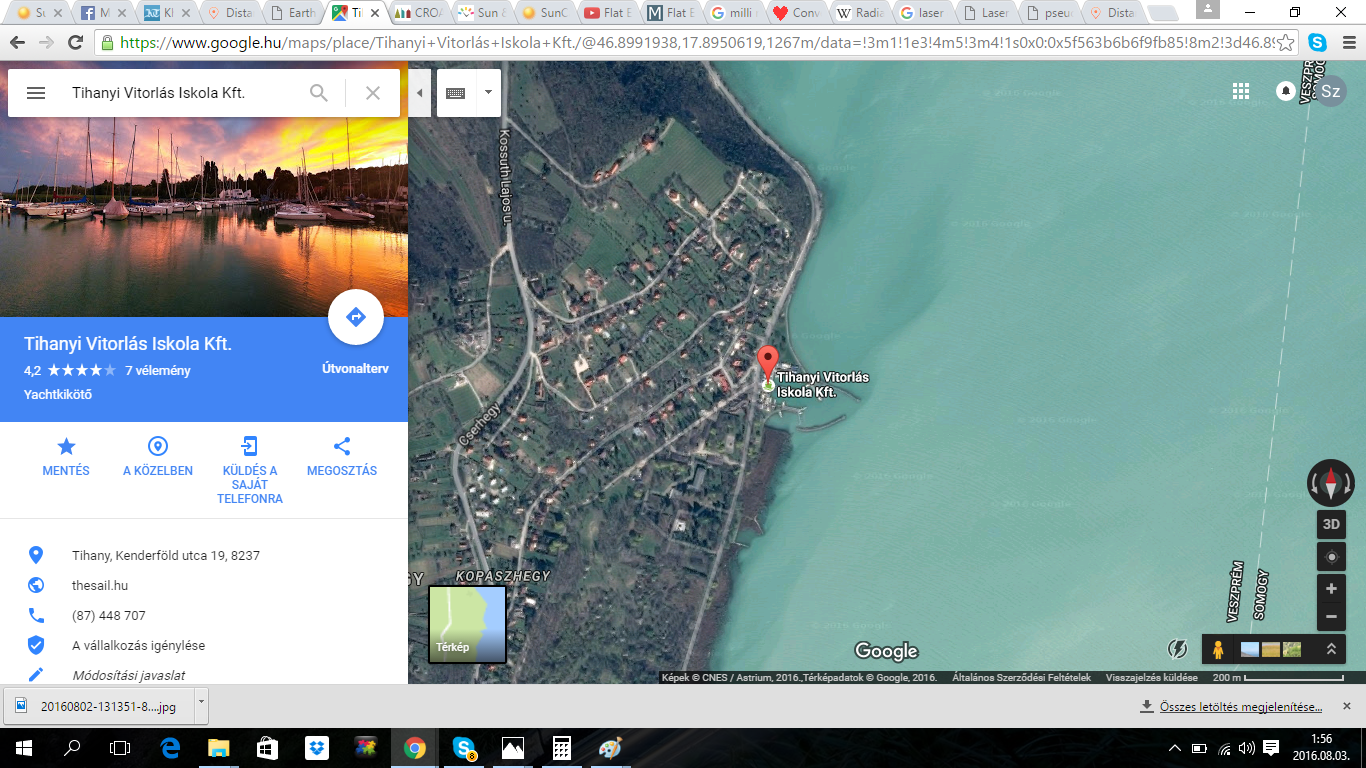here is the completed map, new route in purple: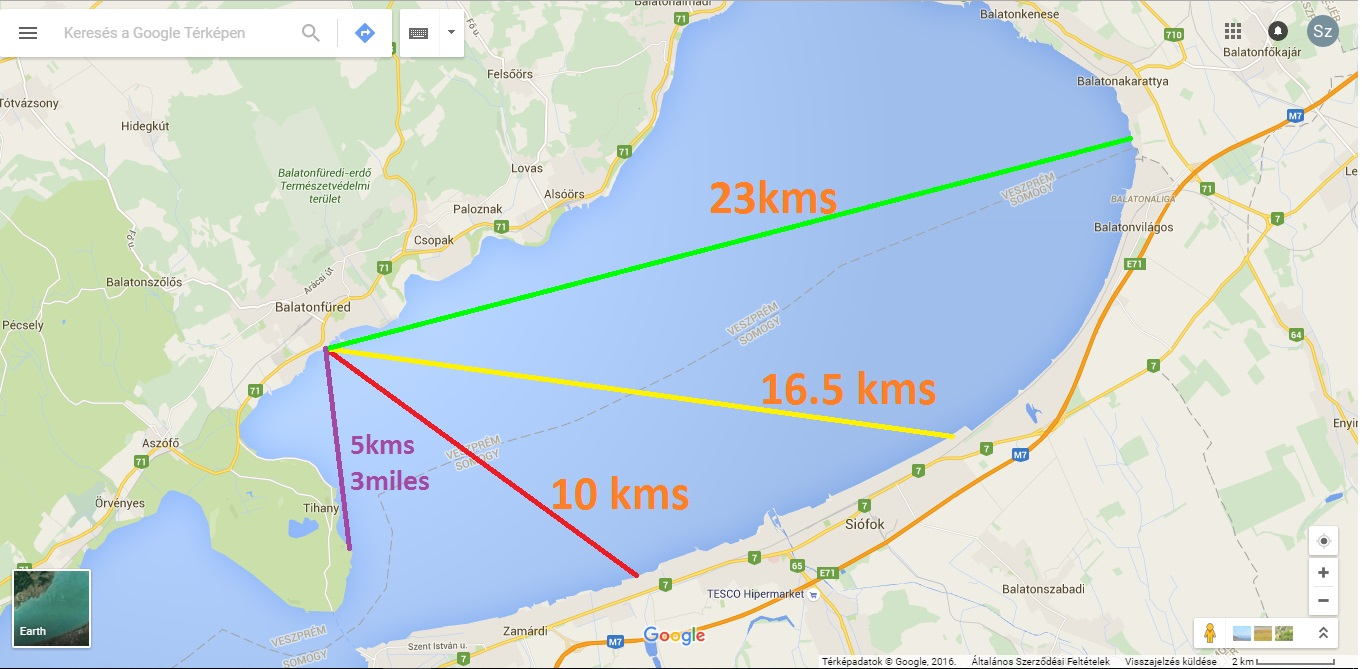#### Chew

##### Senior Member.
How are you going to measure the distances?

#### Sandor Szekely

##### Banned
Banned
How are you going to measure the distances?

Good question thanks! this is very important to define correctly.

We will use a GPS designed to show coordinates on the water so that will be recorded with a camera at each measurement.
I will also show objects and landmarks around with the camera for refrerence point.
We will arrive to locations that we can exactly define on the map and also prove them with camera views.

I am planning to use a teleobjective camera in position A at the laser (same height) FILM WITHOUT CUT! all the way from the start to the end
a teleobjective camera at position B wher the boat is heading to at the exact measurement the same WITHOUT CUT
a gopro camera to record the journey of the boat also without cut

and I have a hand camera to record the measurements and the laser beam direct hit as well.
we will havecamera in position A to record what is happening there
we will have even more cameras you can give ideas where to place them.

PLS tell me if this is correct or you'd like to see something else to define the location exactly.

#### Mick West

Staff member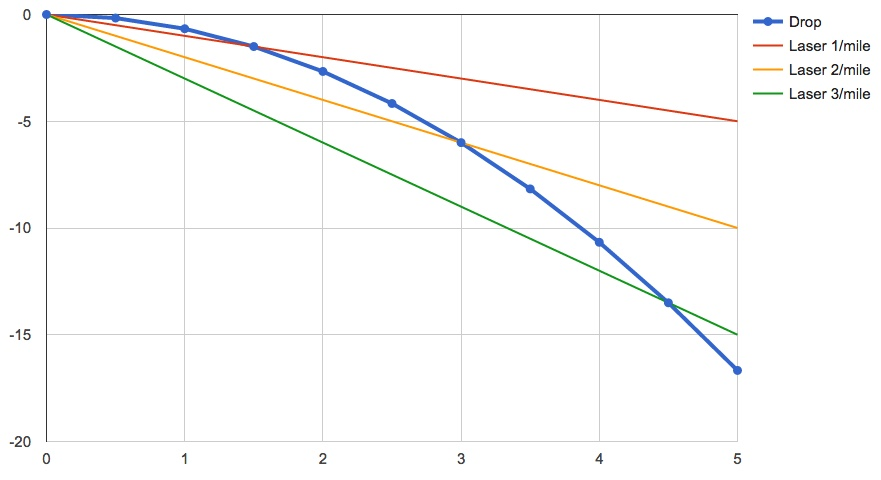The above graph illustrates the problem with a laser that points downwards slightly. The axes units are miles on the horizontal and feet on the vertical, so it represents a greatly compressed view on the actual curve.

So the top of the graph is horizontal. i.e. it's what the laser SHOULD shine along.

The blue line is the curve of the earth. It drops, so it's six feet down at 3 miles, as you would expect, and -17 at 5 miles.

Now if the laser is perfectly horizontal, then the six feet drop will be readily apparent at 3 miles.

However, if the laser dips down a very small amount, just 1 foot per mile (the red line), then the curve will be very hard to detect for the first 2 miles. then at 3 miles it's only dropped 1.5 feet.

If it drops 2 feet per mile (yellow line) then the initial deviation is more apparent (the laser will dip first, then rise up), but at 3 feet it will be back where it started, and you might think the earth was flat if that's all you measured.

If it drops 3 feet per mile then the laser will sink for the first 2.25 miles, and get back to its starting height at 4.5 miles.

Hopefully you can see the problem here. Over certain ranges, a slight slope of the laser will give values that (given the low measuring accuracy) will be very similar to the curve of the earth, and so will cancel it out, making the earth seem flat if you just look at that region of the curve (say, from 4 to 5 miles for the green line drop of 3 feet per mile)

So to counter this, it is very important to get as many readings as possible so you can plot them on a graph, and then quite simply visually see if the graph is a curve or a straight line. If you can visually detect a curve, then you can calculate the slope error (if any) and then the rough radius value.

#### Sandor Szekely

##### Banned
BannedThe above graph illustrates the problem with a laser that points downwards slightly. The axes units are miles on the horizontal and feet on the vertical, so it represents a greatly compressed view on the actual curve.

So the top of the graph is horizontal. i.e. it's what the laser SHOULD shine along.

The blue line is the curve of the earth. It drops, so it's six feet down at 3 miles, as you would expect, and -17 at 5 miles.

Now if the laser is perfectly horizontal, then the six feet drop will be readily apparent at 3 miles.

However, if the laser dips down a very small amount, just 1 foot per mile (the red line), then the curve will be very hard to detect for the first 2 miles. then at 3 miles it's only dropped 1.5 feet.

If it drops 2 feet per mile (yellow line) then the initial deviation is more apparent (the laser will dip first, then rise up), but at 3 feet it will be back where it started, and you might think the earth was flat if that's all you measured.

If it drops 3 feet per mile then the laser will sink for the first 2.25 miles, and get back to its starting height at 4.5 miles.

Hopefully you can see the problem here. Over certain ranges, a slight slope of the laser will give values that (given the low measuring accuracy) will be very similar to the curve of the earth, and so will cancel it out, making the earth seem flat if you just look at that region of the curve (say, from 4 to 5 miles for the green line drop of 3 feet per mile)

So to counter this, it is very important to get as many readings as possible so you can plat them on a graph, and then quite simply visually see if the graph is a curve or a straight line. If you can visually detect a curve, then you can calculate the slope error (if any) and then the rough radius value.

GREAT explanation!

SO we could pick the setup we planned for the experiment:

we will be pretty close to the RED line:
"However, if the laser dips down a very small amount, just 1 foot per mile (the red line), then the curve will be very hard to detect for the first 2 miles. then at 3 miles it's only dropped 1.5 feet."

we can DEFINE here at WHAT DISTANCE? I should make the SLOPE CORRECTION?
my plan was at 2kms that is 1.24 miles and to make it at 50 cms (1.6 feet)

my suggession here is TO USE IMPERIAL UNITS ONLY

SO shall I make it at exactly 1 miles out? or 1.5? What is your suggestion?

the DROP is 0.666 feet (what a mansonic number lol) at 1 miles

that means if I set the beam slope correction to 1.6 feet than I corrected 0.666 feet dip downwards
this makes it a line a bit higher than the RED line
this "RED" line would make the 14.3 miles measurement possible ONLY on FE that PROVES that there is no curvature on water surface.

the Yellow line representing the 2 feet drop is what we will see if the Balaton lake has a curvature like on the globe earth model. We set the beam slope corrected at 1 miles for example to 1.6 feet height, than come back to the starting point, start on the distance and experience a drop in the laser height above water at the first part and then experience a RISE on the beam after a distance. this PROVES curvature on water surface ( not like Hawking's measurement)

the GREEN line is not possible to set as the laser beam hits the surface of the water at a short distance.

"So to counter this, it is very important to get as many readings as possible so you can plat them on a graph, and then quite simply visually see if the graph is a curve or a straight line. If you can visually detect a curve, then you can calculate the slope error (if any) and then the rough radius value."

Mick that's correct.
AND I will do as you said before

go out to the 1 mile distance and make the slope correction
come back to the start point and take measurements rapidly (or possibly continuosly) and when I reach let's say 3 miles distance than we take the measurements every 1/2 mile all the way on the distances
we record this from 3 cameras without cut and record the GPS positions and the surrounding land marks as well

so PLS tell me your opinion on the exact setup for the slope correction distance in imperial values?

#### Mick West

Staff member
so PLS tell me your opinion on the exact setup for the slope correction distance in imperial values?

The exact setup should simply be:
1. Try to get the laser as horizontal as possible
2. Leave it at that setting for the duration of the experiment
3. Take as many readings as possible with accurate height and distance readings (use GPS for distance)
4. Plot the results on a graph of height against distance
5. AFTER, calculate the value of the laser slope correcton, and the curve of the earth (if any)
You want to get the laser as horizontal as possible as that will show any curvature most clearly. However if it's not perfectly horizontal, then you can still get good results providing you take lots of readings.

#### Mick West

Staff member
go out to the 1 mile distance and make the slope correction
come back to the start point and take measurements rapidly (or possibly continuosly) and when I reach let's say 3 miles distance than we take the measurements every 1/2 mile all the way on the distances
we record this from 3 cameras without cut and record the GPS positions and the surrounding land marks as well

The slope correction is not something you do in the field. It's something you to do afterwards, to correct for any slope. You still want to get the laser as horizontal as possible.

If you were to go out to 1 mile, you could do a manual adjustment in two ways.

A) You could set it to the same level as the laser, which will give you something close to the red line error.
B) You could set it to 8 inches ABOVE this level, which will give you an actual horizontal laser (roughly, but better than 30 feet from shore)

You can do either of these. Option B would be preferable, but option A would work, providing you made enough readings to discern if there was a curve in the graph that the readings form.

If you believe in the Flat Earth theory, then you might think that option B is "rigging" the experiment by making the laser point up in the air. However this would be revealed by the curve of the graph, and by the analysis I described in this post:
https://www.metabunk.org/lake-balat...-of-the-earth-if-any.t7780/page-2#post-186851

And note that regardless of if you choose A) or B), there will almost certainly be a slope to the laser line, but the math will figure that out after.

Last edited:

#### Sandor Szekely

##### Banned
Banned
The exact setup should simply be:
1. Try to get the laser as horizontal as possible
2. Leave it at that setting for the duration of the experiment
3. Take as many readings as possible with accurate height and distance readings (use GPS for distance)
4. Plot the results on a graph of height against distance
5. AFTER, calculate the value of the laser slope correcton, and the curve of the earth (if any)
You want to get the laser as horizontal as possible as that will show any curvature most clearly. However if it's not perfectly horizontal, then you can still get good results providing you take lots of readings.

Mick we agreed that I am not setting laser beam to horizontal but a "slope corrected" angle

I see here that the term "slope corrected" is not exact to replace "laser leveling"

please decribe this what we do here:
1. we "level" the laser to 1.6 feet above water surface at a distance of 1 miles - the same height as the laser beam is a the starting point

SO this is our PRE defined slope correction : we can calculate with this and we will set this exactly at the measurement

with all other points I agree#### Mick West

Staff member
Also you might want to figure out some way of adjusting the laser beyond the rough adjustments of adding plastic sheets underneath it. Some kind of screw adjustment.

#### Sandor Szekely

##### Banned
Banned
The slope correction is not something you do in the field. It's something you to do afterwards, to correct for any slope. You still want to get the laser as horizontal as possible.

If you were to go out to 1 mile, you could do a manual adjustment in two ways.

A) You could set it to the same level as the laser, which will give you something close to the red line error.
B) You could set it to 8 inches ABOVE this level, which will give you an actual horizontal laser (roughly, but better than 30 feet from shore)

You can do either of these. Option B would be preferable, but option A would work, providing you made enough readings to discern if there was a curve in the graph that the readings form.

If you believe in the Flat Earth theory, then you might think that option B is "rigging" the experiment by making the laser point up in the air. However this would be revealed by the curve of the graph, and by the analysis I described in this post:
https://www.metabunk.org/lake-balat...-of-the-earth-if-any.t7780/page-2#post-186851

And note that regardless of if you choose A) or B), there will almost certainly be a slope to the laser line, but the math will figure that out after.

I would do A) as it is the right measurement to find water level flat. This setup will show if there is curvature on water at a 6+ miles distance and it can be measured all the way to the 14.3 miles only if the water is absolutely flat.

"there will almost certainly be a slope to the laser line, but the math will figure that out after."

this is correct!

Last edited:

#### Mick West

Staff member
Mick we agreed that I am not setting laser beam to horizontal but a "slope corrected" angle

I see here that the term "slope corrected" is not exact to replace "laser leveling"

please decribe this what we do here:
1. we "level" the laser to 1.6 feet above water surface at a distance of 1 miles - the same height as the laser beam is a the starting point

SO this is our PRE defined slope correction : we can calculate with this and we will set this exactly at the measurement

with all other points I agreeI think there's a little miscommunication here. Ideally you want the laser beam to be horizontal. i.e. at right angles to vertical (where vertical is defined by gravity). So you want the laser to be level, like a carpenter's level would indicate.

Now this is a hard to do with a short level and have it accurate over 5 miles. So adjusting it at 1 mile is a good idea.

However the way you adjust it has different meanings depending on if the Earth is flat or not.

A) If you think the earth is flat you set it 1.6 feet (same as laser at start) to get horizontal. However if the earth is round then this introduces an error (red line in the graph above). It still works fine if you do the math, but is less accurate.

B) If you think the Earth is round, then you'd adjust it to 1.6 + 0.66 = 2.26 feet, and this will give you a perfectly horizontal laser, and the clearest results. However if the Earth is flat, then this will make the laser rise up with distance, linearly.

But like I said, it does not matter which one you do, providing you take lots of readings. Since the Earth actually IS round, then option B is the most accurate. However I can understand the preference for A (and suspicion regarding B), if you actually think the earth is flat. So just pick one.

Staff member

#### Sandor Szekely

##### Banned
Banned
I think there's a little miscommunication here. Ideally you want the laser beam to be horizontal. i.e. at right angles to vertical (where vertical is defined by gravity). So you want the laser to be level, like a carpenter's level would indicate.

Now this is a hard to do with a short level and have it accurate over 5 miles. So adjusting it at 1 mile is a good idea.

However the way you adjust it has different meanings depending on if the Earth is flat or not.

A) If you think the earth is flat you set it 1.6 feet (same as laser at start) to get horizontal. However if the earth is round then this introduces an error (red line in the graph above). It still works fine if you do the math, but is less accurate.

B) If you think the Earth is round, then you'd adjust it to 1.6 + 0.66 = 2.26 feet, and this will give you a perfectly horizontal laser, and the clearest results. However if the Earth is flat, then this will make the laser rise up with distance, linearly.

But like I said, it does not matter which one you do, providing you take lots of readings. Since the Earth actually IS round, then option B is the most accurate. However I can understand the preference for A (and suspicion regarding B), if you actually think the earth is flat. So just pick one.

I fully understand and aggree with you on this!

"Ideally you want the laser beam to be horizontal. i.e. at right angles to vertical "

no misscomunication on thisI am setting an exact angle very close to horizontal. With the setup we fixed at 1 miles out same height as the orig point it is a minimal degree deviation downwards (red line) on the globe model and 0 on the flat model.
So I like this, because it is well provable#### Mick West

Staff member
I am setting an exact angle very close to horizontal. With the setup we fixed at 1 miles out same height as the orig point it is a minimal degree deviation downwards (red line) on the globe model and 0 on the flat model.
So I like this, because it is well provable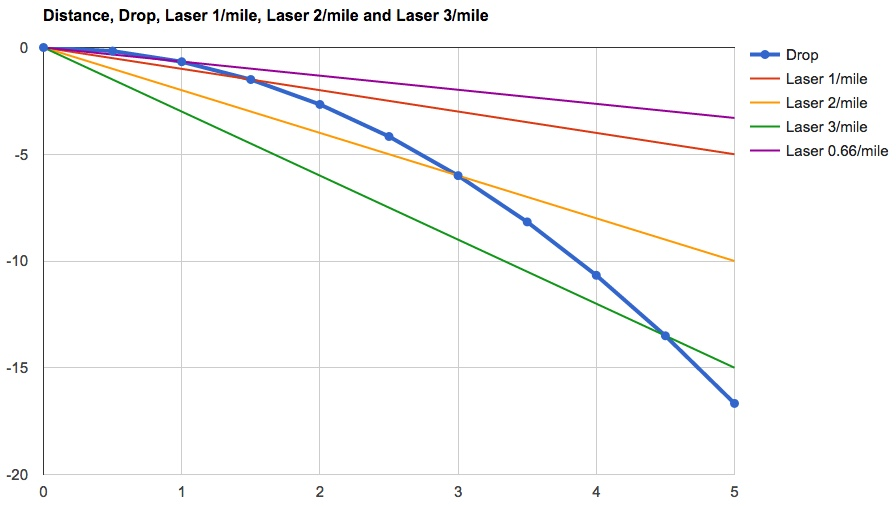If adjusted to the same height as the original at 1 mile you'd get the purple line.

Note that a drop of 0.66 feet per mile is a slope of less than 0.01°, so you can see how hard it would be to have zero slope on the laser, and hence the need for multiple readings.

#### Sandor Szekely

##### Banned
BannedIf adjusted to the same height as the original at 1 mile you'd get the purple line.

Note that a drop of 0.66 feet per mile is a slope of less than 0.01°, so you can see how hard it would be to have zero slope on the laser, and hence the need for multiple readings.

Perfect!

besides the manual height measurements with a floating level meter combined with tape measure, we could make a continuos video measurement like this:

we make the coloured level signals with tape on the white board at the experiment recorded on video in the bay before we make the experiment. We will do it with the same number of people as in the experiment to be exact.
the orange is representing a camera
How does that sound?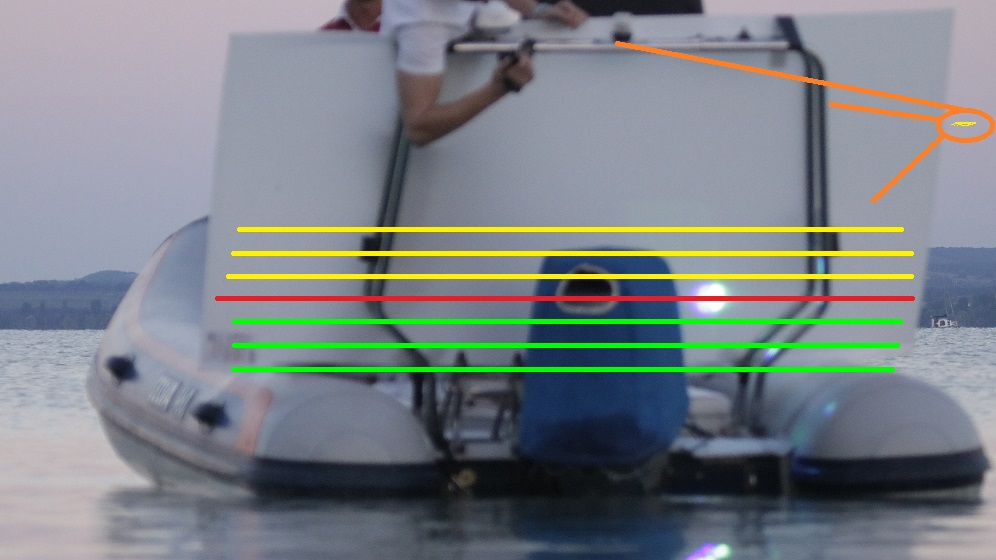#### Mick West

Staff member
Perfect!

besides the manual height measurements with a floating level meter combined with tape measure, we could make a continuos video measurement like this:

we make the coloured level signals with tape on the white board at the experiment recorded on video in the bay before we make the experiment. We will do it with the same number of people as in the experiment to be exact.
the orange is representing a camera
How does that sound?I'm not sure what you would expect this to show?

#### Sandor Szekely

##### Banned
Banned
I'm not sure what you would expect this to show?

the different colour lines will be made with a stick tape to be able to read the height of the laser through a camera continuosly.
we will make measurements as well, but this will show the laser beam level continuosly

#### Chew

##### Senior Member.
We will use a GPS designed to show coordinates on the water...

GPS? GPS displays coordinates according to the spherical earth model. The difference in the length of a degree of longitude between the FE and SE model rapidly increases the farther you get from the North Pole. You can verify GPS uses the SE model by driving on a road that runs exactly east and west and measuring the distance with your car's odometer and comparing the distance as measured by Google Earth (which uses the SE model). If Google Earth and your odometer match then GPS is using the SE model and you'll need to hire a surveyor to measure the distances across the lake because according to the FE theory the distances should not match with the SE model.

#### Mick West

Staff member
GPS? GPS displays coordinates according to the spherical earth model. The difference in the length of a degree of longitude between the FE and SE model rapidly increases the farther you get from the North Pole. You can verify GPS uses the SE model by driving on a road that runs exactly east and west and measuring the distance with your car's odometer and comparing the distance as measured by Google Earth (which uses the SE model). If Google Earth and your odometer match then GPS is using the SE model and you'll need to hire a surveyor to measure the distances across the lake because according to the FE theory the distances should not match with the SE model.

That's really a different topic. I think we can all agree that GPS gives fairly accurate position readings, and over the small scale distances we have here it's quite adequate for determining surface distance between points

#### Sandor Szekely

##### Banned
Banned
I think so too, that on the given distances up to 14.3 miles the difference between the GE and FE model is very little. Indeed we can correct the distances with the curvature math, but the GPS postition will not be as accurate as the correction itself.

#### DarkStar

##### Active Member
GPS distances would be great circle, but yeah -- at 14.3 miles it's a negligible difference.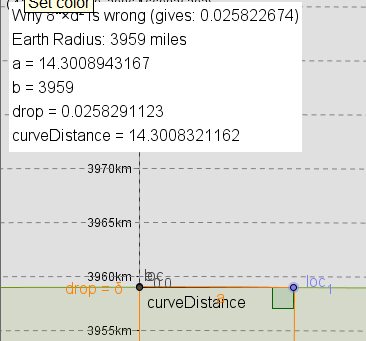my calc, inspired by Mick'sAside: My post on WHY 8"xd² is wrong:
https://flatearthinsanity.blogspot.com/2016/08/flat-earth-follies-how-to-derive-8-per.html

Note that I concede that it is "close enough for government work" for <100 miles.

Just in case anyone cared in this context.

#### DarkStar

##### Active Member
I think so too, that on the given distances up to 14.3 miles the difference between the GE and FE model is very little. Indeed we can correct the distances with the curvature math, but the GPS postition will not be as accurate as the correction itself.

Sandor, you seem pretty reasonable about the math so let me implore you to review:

http://flatearthinsanity.blogspot.com/2016/07/simple-proof-for-convexity-of-earth.html

Here is the heart of the proof.

Simply pick a line of longitude (along which everyone will experience high noon at the same time, doesn't have to be one perfect line of latitude but each person should measure at high noon on the same day) and measure the length of the sun's shadow at several points of latitude from directly under the sun to as far North as you can (must have at least one measurement that is above 75°). [I give a few more details in my post, must be plumb, etc]

That's all you have to do.

You can review my mathematics in more detail but IF we lived on a Flat Earth then, as you moved perpendicular to the sun's path, you would find the lengths of shadows to be proportional to the distance from the subsolar point. On a convexly curved Earth they would get longer as a power curve (which is what we observe), and on a concave Earth they would vary logarithmically.

This is a trivially true observation geometrically and the mathematics are very simple as well. And it doesn't matter what the altitude of the sun actually is! That would affect the ACTUAL values of course, but not the fact that the Gaussian curvature DEFINES the shape of this measurement.

And the cool thing is that twice a year kids around the world perform this measurement so we have years and years of data collected by kids.

http://ciese.org/curriculum/noonday/gallery/

They usually just calculate the circumference but 'hidden' in this data is a very simple, undeniable, geometric truth - the Earth is convexly curved.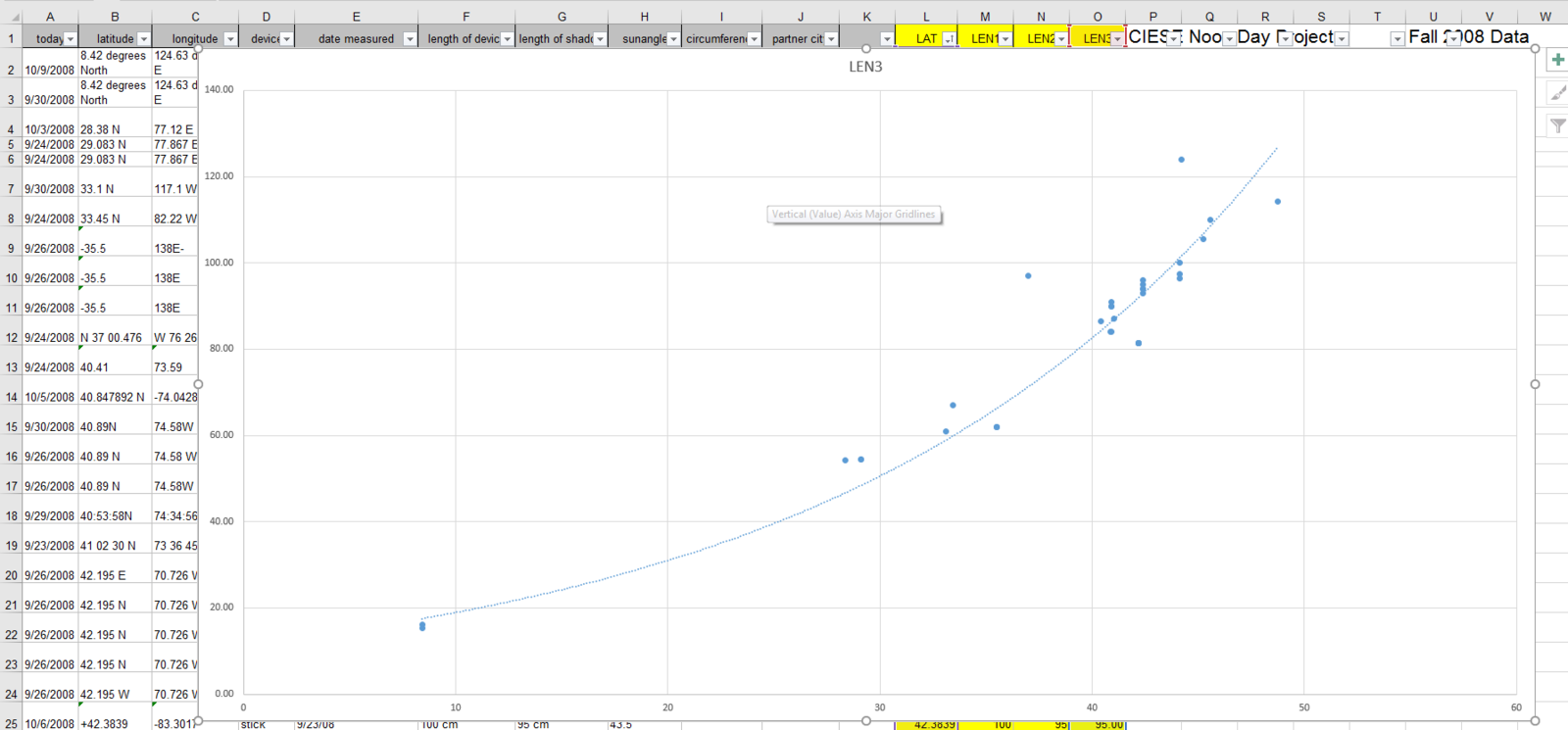Last edited:

#### DougW

##### Member
Sandor - When are you expecting to do your experiment?

#### Sandor Szekely

##### Banned
Banned
Sandor - When are you expecting to do your experiment?

It will be on the 15th / 16th of August ( in 10 days)

#### Chew

##### Senior Member.
I think so too, that on the given distances up to 14.3 miles the difference between the GE and FE model is very little.

What would be the distance according to the flat earth model?

#### Sandor Szekely

##### Banned
Banned
What would be the distance according to the flat earth model?

this is DarkStar's calculation:

+0.0008943167 mile that is 56.66 inches on 14.3 miles meaning a 99.993746428159 % accuracyand this is mine
+0.0001 mile that is 6,336 inches on 14.3 miles ameaning a 99.99930070419088 % accuracy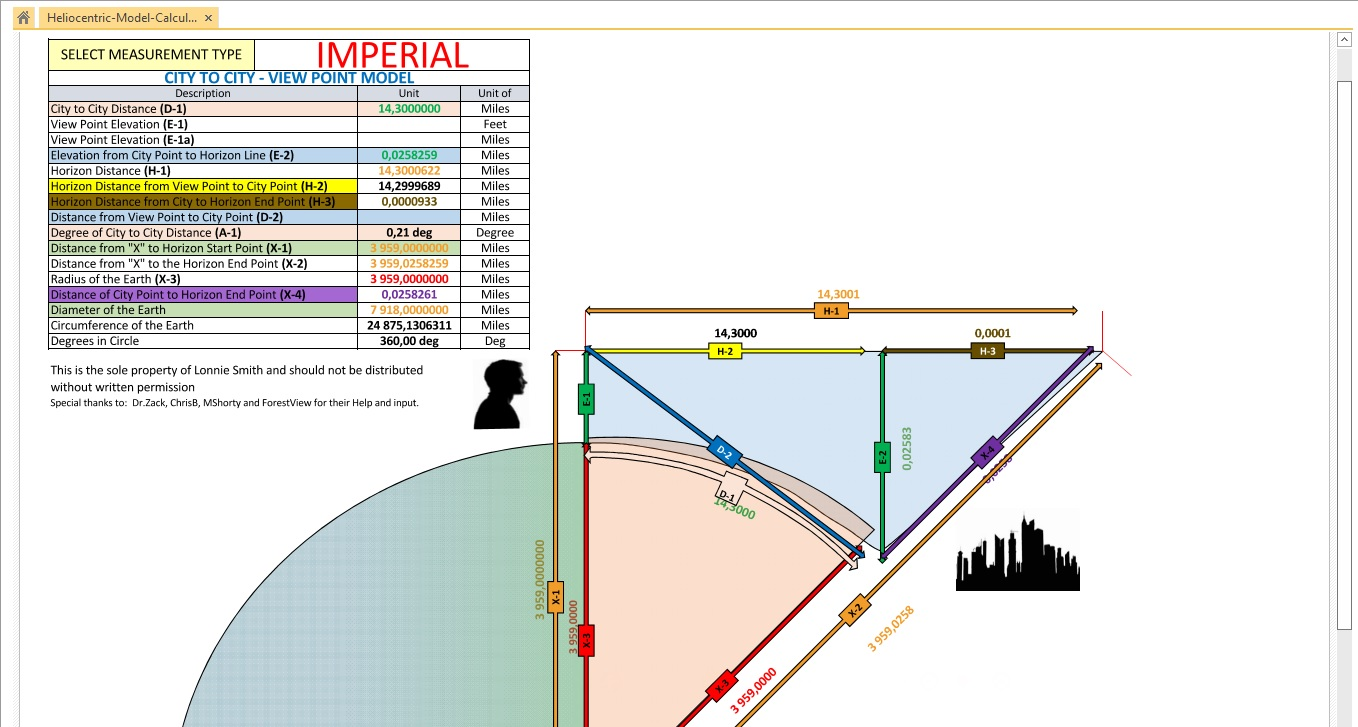marginal difference in both cases

#### Chew

##### Senior Member.
and this is mine
+0.0001 mile that is 6,336 inches on 14.3 miles ameaning a 99.99930070419088 % accuracy

What? That is not how you calculate distances on a flat earth. On a flat earth the length of a degree of longitude is linearly proportional to the distance from the north pole.

For a flat earth with a diameter of 24,857 statute miles a degree of longitude at the circumference of the flat earth is 216.92 statute miles. The length of a degree of longitude is (90° - latitude)/180° * 216.92 statute miles, where southern latitudes are treated as negative numbers.

The length of a degree of longitude on the globe earth is cosine(latitude) * 69.05 statute miles.

At the latitude of your experiment a degree of longitude on a flat earth is 10.5% longer than a degree of longitude on a globe earth.

What is the latitude and longitude of the easternmost point on your map of the lake? I measured a distance of 21.76 km instead of 23 km.

#### Sandor Szekely

##### Banned
Banned
What? That is not how you calculate distances on a flat earth. On a flat earth the length of a degree of longitude is linearly proportional to the distance from the north pole.

For a flat earth with a diameter of 24,857 statute miles a degree of longitude at the circumference of the flat earth is 216.92 statute miles. The length of a degree of longitude is (90° - latitude)/180° * 216.92 statute miles, where southern latitudes are treated as negative numbers.

The length of a degree of longitude on the globe earth is cosine(latitude) * 69.05 statute miles.

At the latitude of your experiment a degree of longitude on a flat earth is 10.5% longer than a degree of longitude on a globe earth.

What is the latitude and longitude of the easternmost point on your map of the lake? I measured a distance of 21.76 km instead of 23 km.

We are not placing our measurement on the flat earth map, we are measuring a water surface horizontal straightness over a lng distance. As we calculated the measurement will be 99.99% to 99.999% accurate on distances.

"What is the latitude and longitude of the easternmost point on your map of the lake? I measured a distance of 21.76 km instead of 23 km."

Very well pointed out Thanks!

Our first setup was like position A laser at the east side and the laser beam poinitng to the bay of Tihany
that is exactly 14.3 miles distance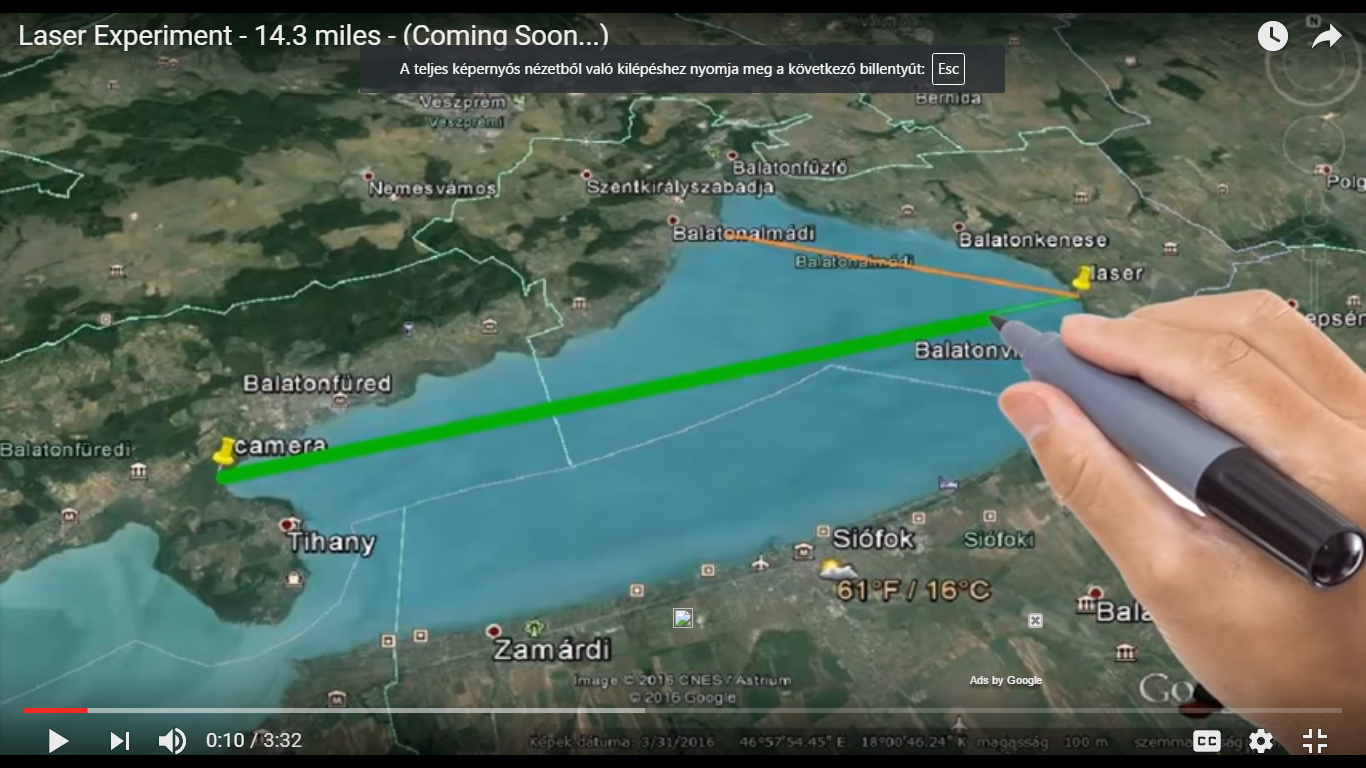original teaser video here:

But we changed the setup to place position A laser to Balatonfured therefore the distande CHANGED

your distance measurement is pretty accurate, we will exactly define distance on the measurement GPS data

here is position A laser ( on the shore of course)

46°56'56.24"N
17°53'22.47"E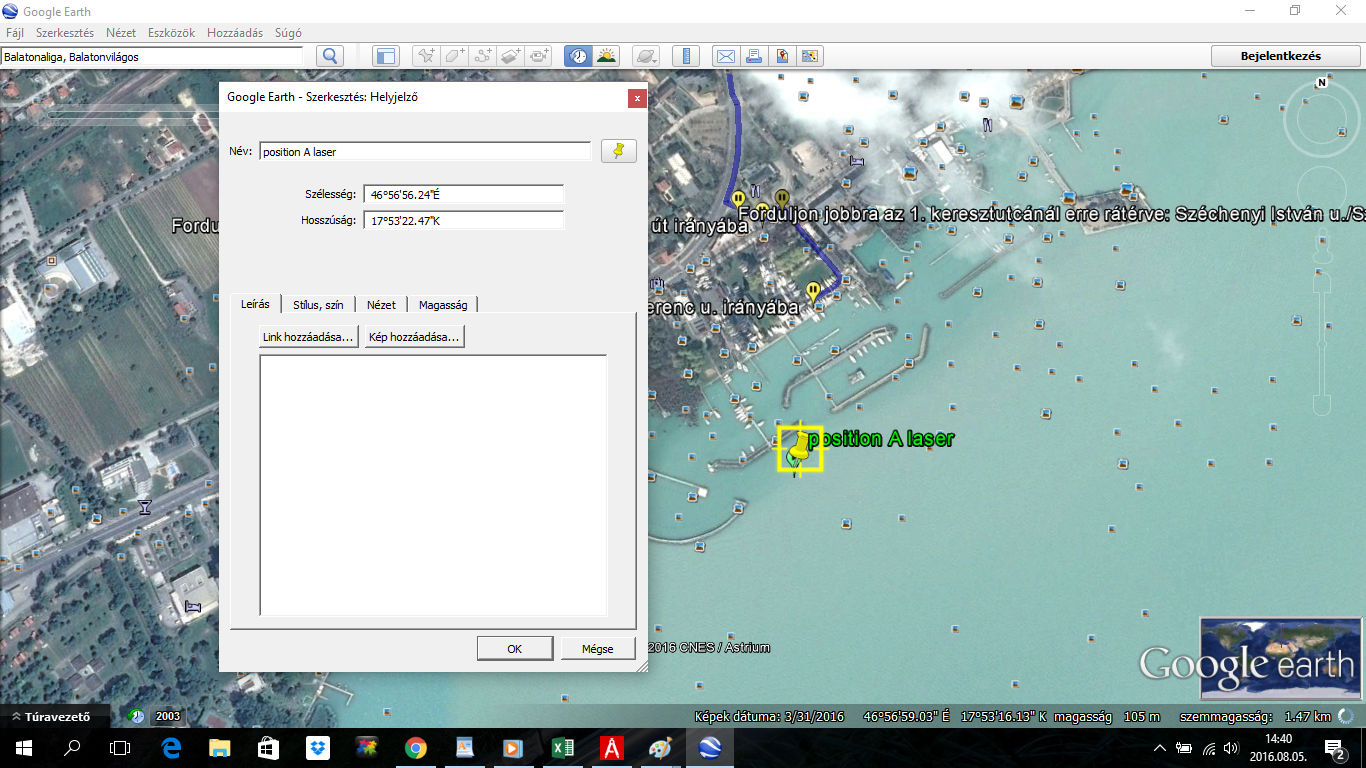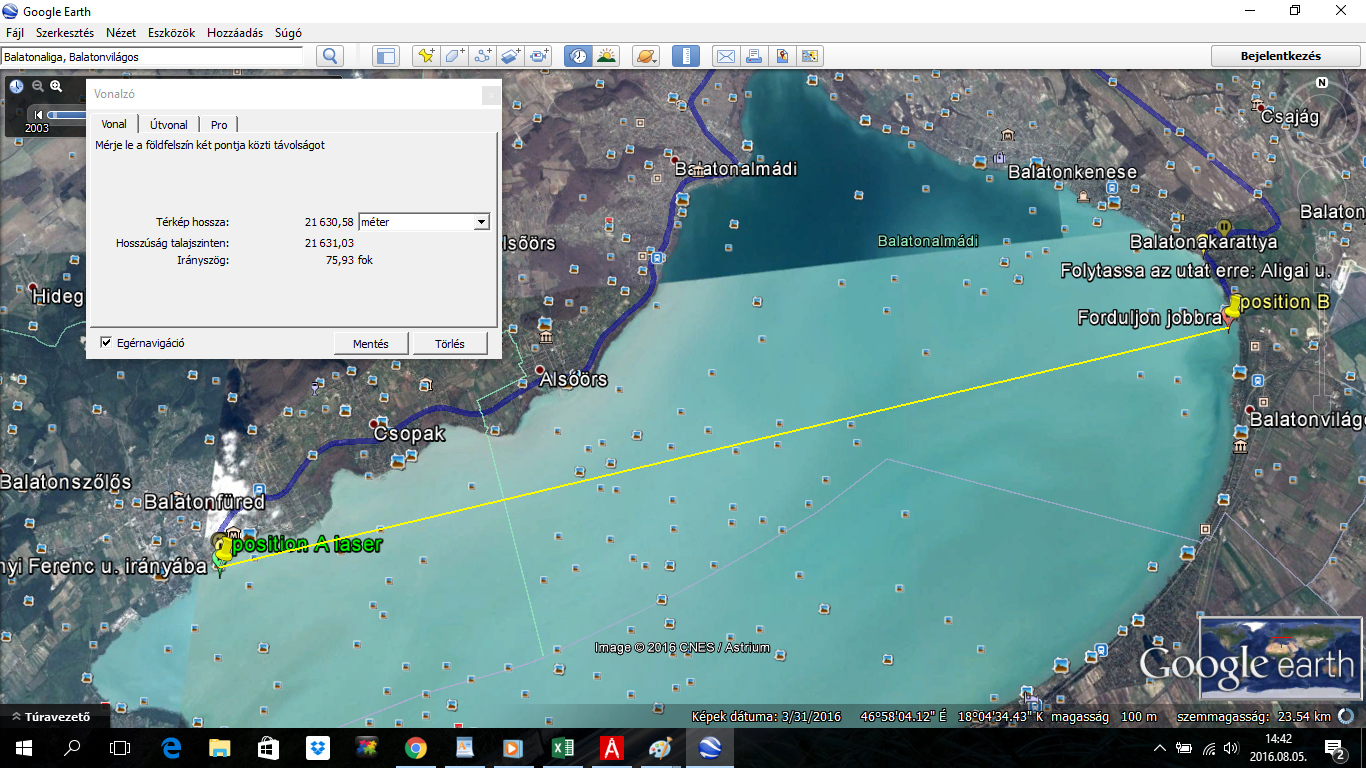SO we will change the title according to the measured distance#### DarkStar

##### Active Member
What? That is not how you calculate distances on a flat earth. On a flat earth the length of a degree of longitude is linearly proportional to the distance from the north pole...

Hi Chew,

I'm not sure what the objection is to the distance calculation if we can measure the distance in Google Earth and the answer is 99.99% the same as flat earth for this distance.

For my example I used a Google Earth reading of 14.3008321162 miles as the great circle distance.

If you wanted the straight-line distance for that it would be 14.3008943167

So we're talking about a difference of 0.0000622005 miles or 3.941 inches -- so who cares? There is no possible way you can measure that distance accurately anyway. This is a short enough distance that we just don't need to care how we measure the distance so long as we all agree that Google Earth distance would be 'good enough'.

Where I have a HUGE issue with the approach is what was discussed above -- leveling the laser.

WHY NOT LEARN FROM THE LESSON OF WALLACE?

Put the laser on one end and standards at the far end and in the middle, with a target at a known height above the water -- point the laser center target on the FAR standard and then see where it hits the middle standard.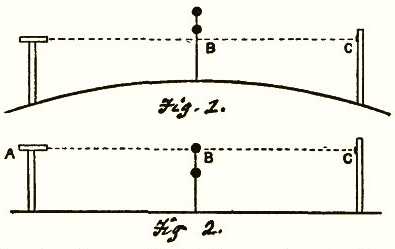If it hits below the target on the middle standard then the water is clearly curved - period. No BSing around with complex slope+curvature calculations or leveling procedures or any other nonsense that is just going to add confusion to the results.

Someone want to calculate the ANGLE at which the laser would be 'tilted' for a 14 mile shot? I think you'd need to be at about 85 feet elevation to hit the distant targetat 73920 feet (14 miles) you should have a rise of 130.7 feet, so that's 0.10076 degrees top to bottom, so would be pretty slight.

#### Mick West

Staff member
WHY NOT LEARN FROM THE LESSON OF WALLACE?

Put the laser on one end and standards at the far end and in the middle, with a target at a known height above the water -- point the laser center target on the FAR standard and then see where it hits the middle standard.If it hits below the target on the middle standard then the water is clearly curved - period. No BSing around with complex slope+curvature calculations or leveling procedures or any other nonsense that is just going to add confusion to the results.

I've suggested several times to @Sandor Szekely, that it would be far better to use a fixed point (like a headland) in the middle and end, rather than a boat. Then accurate measurements could be taken. But he prefers the boat.

The calculations are a little complex, however you don't need them. You just need to show that the measurements do not lay on a straight line. The Wallace experiment demonstrates this with three points. Since Sandor is using a boat I've suggested that he takes as many measurements as possible so the curve will show through the noise.

The Wallace setup at 3 miles would be the same as the orange line here:With the laser tilted down at 2 feet per mile.

Sandor has indicated he's going to do something more like the purple line (0.66 feet per mile, by leveling the laser at 1 mile. This will give a greater (and more apparent) deviation at 3 miles than the Wallace method of leveling at 3 miles, and then going back to the center.

#### Chew

##### Senior Member.
I'm not sure what the objection is to the distance calculation if we can measure the distance in Google Earth

Using Google Earth is the problem. It calculates the distance between sets of lat and long using a spherical model of the earth. As I pointed out the lengths of a degree of longitude vary greatly between the two models.

If the coordinates are correct and the earth is flat then the distance must be wrong. If the distance is correct and the earth is flat then the coordinates must be wrong.

If both the coordinates and the distance is correct then the earth is a sphere.

#### Mick West

Staff member
Using Google Earth is the problem. It calculates the distance between sets of lat and long using a spherical model of the earth. As I pointed out the lengths of a degree of longitude vary greatly between the two models.

If the coordinates are correct and the earth is flat then the distance must be wrong. If the distance is correct and the earth is flat then the coordinates must be wrong.

If both the coordinates and the distance is correct then the earth is a sphere.

That's a different issue though. We know there's many such inconsistencies in the Flat Earth model, but can we just accept that the distances GPS gives between any two points are accurate, and have Sandor do his laser experiment?

#### Chew

##### Senior Member.
but can we just accept that the distances GPS gives between any two points are accurate

I do accept the distances are accurate. I'm just baffled as to why Sandor accepts it.

#### Mick West

Staff member
I do accept the distances are accurate. I'm just baffled as to why Sandor accepts it.
Well that might be a good topic for another thread. Perhaps with some example road-trip experiments that people might do to test it.

#### Sandor Szekely

##### Banned
Banned
Where I have a HUGE issue with the approach is what was discussed above -- leveling the laser.

WHY NOT LEARN FROM THE LESSON OF WALLACE?

Put the laser on one end and standards at the far end and in the middle, with a target at a known height above the water -- point the laser center target on the FAR standard and then see where it hits the middle standard.If it hits below the target on the middle standard then the water is clearly curved - period. No BSing around with complex slope+curvature calculations or leveling procedures or any other nonsense that is just going to add confusion to the results.

Someone want to calculate the ANGLE at which the laser would be 'tilted' for a 14 mile shot? I think you'd need to be at about 85 feet elevation to hit the distant targetat 73920 feet (14 miles) you should have a rise of 130.7 feet, so that's 0.10076 degrees top to bottom, so would be pretty slight.

I understand your point on the Wallace setup, you'd like to measure the saggita height of the curvature on the given distance.

I am ready to make measurement dedicated to Metabunk ideason the experiment day, especially at daytime when our laser will not be well seen on long distance. Sunset, night time and sunrise will be dedicated to the long range measurements, and I suggest the morning daytime on the reason of less refraction that we talk about in the other post (in Jeranism's video).

I CAN find a proper target on land and calculate the height from the water surface in position B.
Indeed I have concerns with the measurement accuracy due to smaller changes to measure.

Like the purple line? OR ELSE?We will see the expected results of the globe model to see if this measurement CAN BE? accurate enough.

"complex slope+curvature calculations or leveling procedures or any other nonsense that is just going to add confusion to the results."
I will do the "original" long range experiments as well, and I think we have figured out a GOOD explanation and calculation method to the long range measurements.

The long range measurements are giving a HUGE difference on the GE and FE models! THERE IS NO DOUBT ABOUT THE OUTCOMEAs Mick said with our setup of leveling the laser as agreed upon (1 miles out) we can not measure the height of the beam after 5 miles or so. That is a pretty good well presentable result.

Here is the calculation of the 14.3 miles distance ( we will correct this to the exact measurement distances)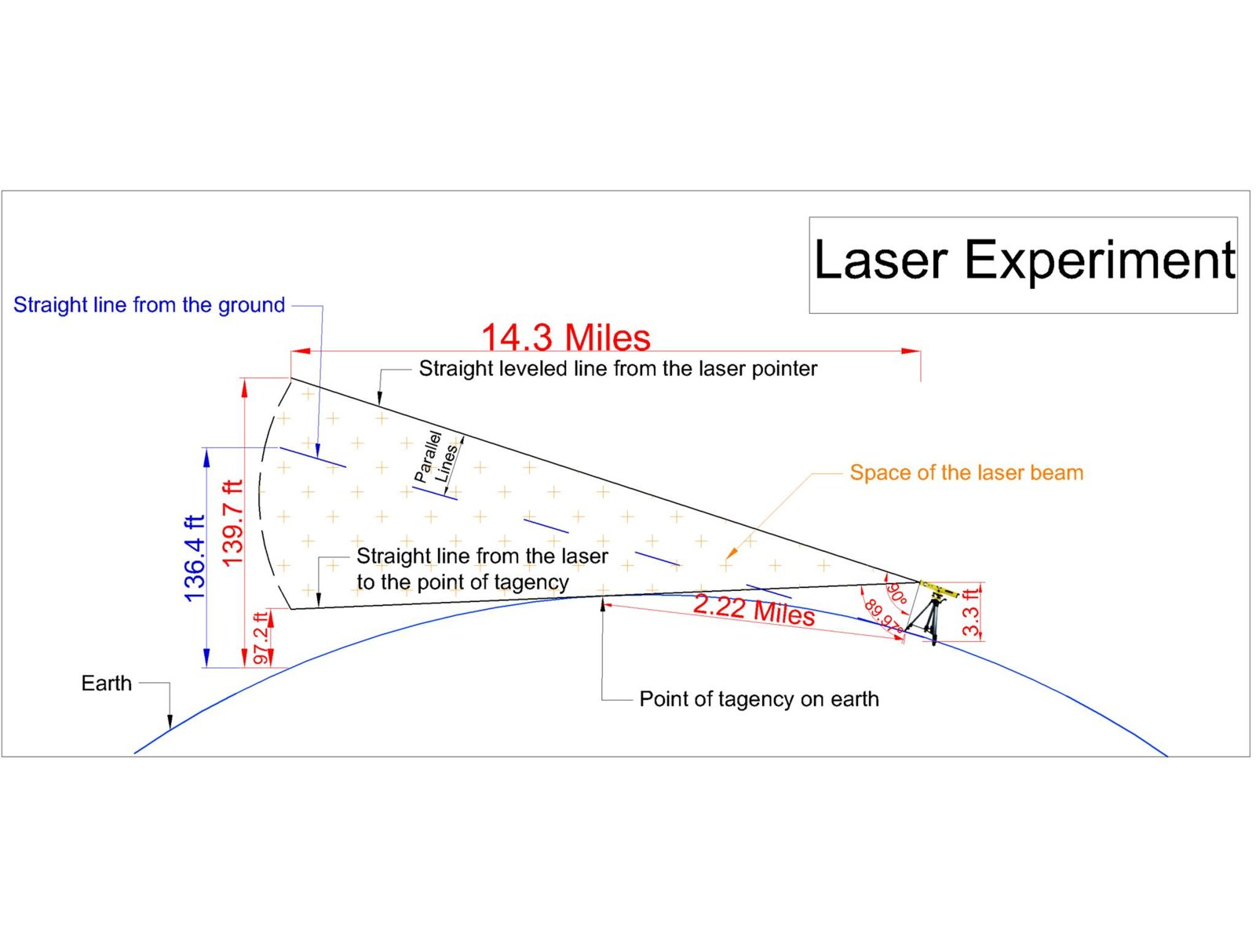WHY I USE A BOAT?The main reasons for the boat is the laser beam height measurement on the complete distance. We can set many points to define the line of the laser beam and also to have reference points for the refraction arguments.
We have a much better way of leveling the laser to the 0.66 feet / mile slope corrected height, AND we can follow the beam to the other side so we don't have a hard time finding it (houses and beaches all around the lake).
It looks cool and it is used in Hawking's film too (we will have a helicopter measurement over land after this).

I'd like to point out, that there is an airfield about 1 km from the position B at the 13.4 miles measurement and I have a pilot with an MI-8 helicopter ready if I need him on this measurement!

DO I NEED a helicopter in your opinion to prove this subject better?
Hawking had it in his video, I am not sure if it is a DEFINITE proof or not.

Status
Not open for further replies.

Replies
19
Views
3K
Replies
57
Views
3K
Replies
4
Views
2K
Related Articles